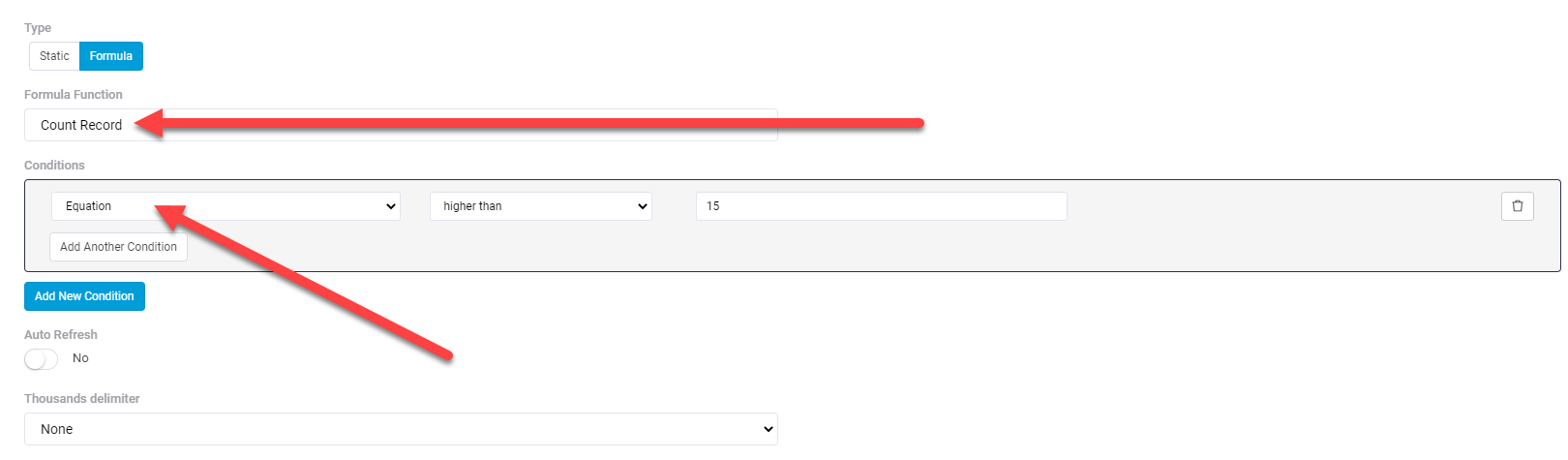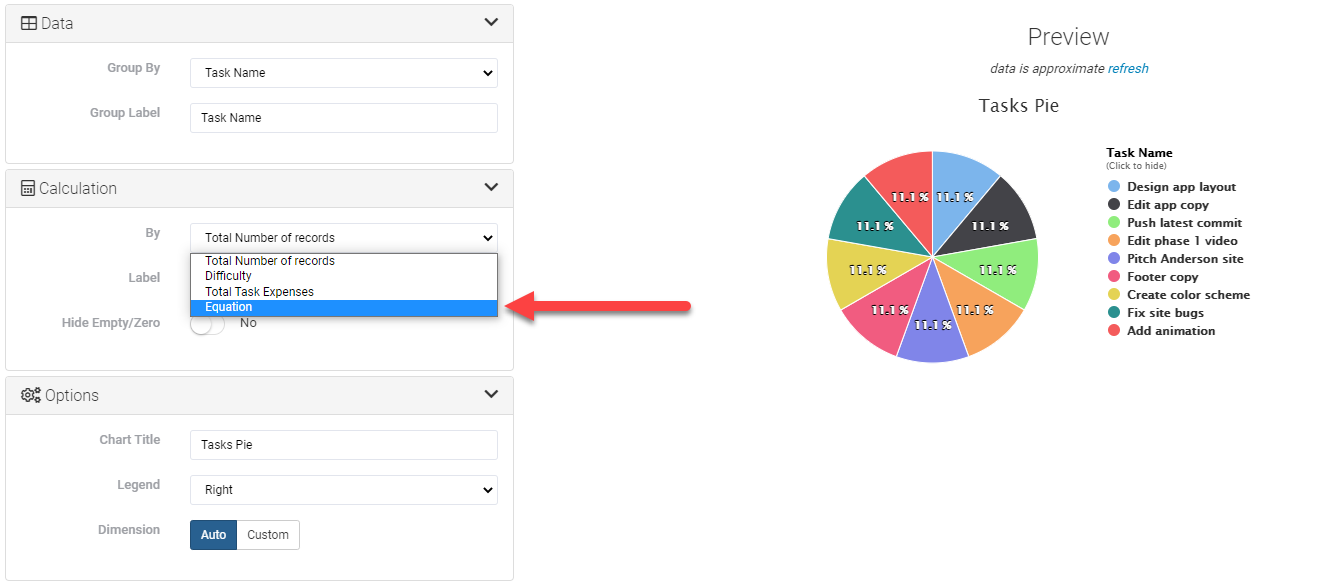# Add equation fields in Cards and Charts

In our most recent update, you can now use Equation Fields as Sum or Average functions in Cards and Charts.

#### Cards

In the Cards component, select your Equation field when using the Sum or Average formula function, and set conditions based on the Equation field.#### Charts

In the Charts component, select your Equation field in the Calculation section to display data in your Chart based on the Equation field.